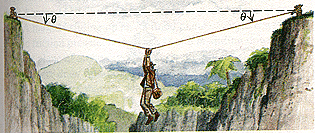generals336

2021-02-20

An adventurous archaeologist crosses between two rock cliffs by slowly going hand-over-hand along a rope stretched between the cliffs. He stops to rest at the middle of the rope (Fig. 1) The rope will break if the tension in it exceeds $4.50\cdot {10}^{4}$N and our hero's mass is 86.5 kg.Figure 1 a) If the angle $\theta$ is ${13.0}^{\circ }$, find the tension iin the rope.

b) What is the smallest value the angle $\theta$ can have if the rope is not to break?krolaniaN

Expert

Each half of the rope exerts a vertical force of $T\cdot \mathrm{sin}\left(\theta \right)$
or $2\cdot T\cdot \mathrm{sin}\left(\theta \right)=M\cdot g$
So
For the minimum angle $\mathrm{sin}\left(\theta \right)=M\cdot \frac{g}{2}\cdot T$
So $\theta$ minimum =.54deg

Do you have a similar question?